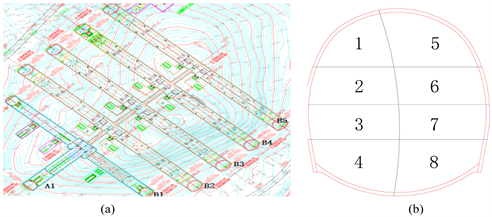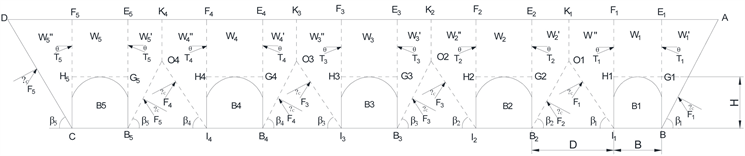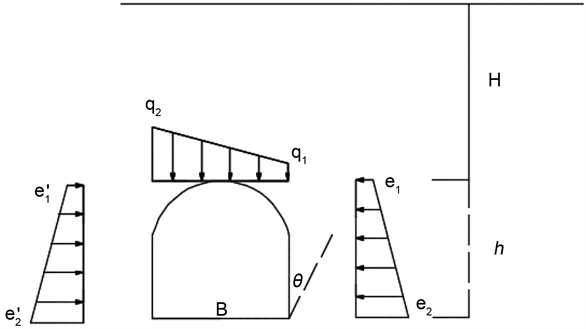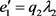﻿ 腾讯七星数据中心隧道群围岩压力分析

# 腾讯七星数据中心隧道群围岩压力分析Analysis on Surrounding Rock Pressure of Tencent Seven Star Data Center Tunnel Group

Abstract: Taking Guiyang Tencent Seven Star Data Center Tunnel Project as the research background, the project belongs to the construction of five closely spaced parallel, shallow, large-section tunnel group, which is the first case at home. The CD method is used in the place where the tunnel geo-logical conditions are good, and the poor place is adjusted to the CD method and step method con-struction. The tunnel group adopts the method of synchronous propulsion and synchronous exca-vation. Due to the stress superposition and the surrounding rock stress concentration caused by the excavation of adjacent tunnels, by establishing the tunnel group pressure calculation model, and using the Rankine stress theory and the Bill Bowman theory to calculate the horizontal side pressure and vertical pressure of the tunnel excavation surrounding rock, the distribution char-acteristics of tunnel surrounding rock pressure and the subsidence of the dome are analyzed. The minimum support reaction force of the tunnel is calculated. The research results can provide ref-erence for tunnel support design.

1. 引言

2. 工程概况Figure 1. Overview of tunnel engineering. (a) Tunnel group plan, (b) Tunnel CD construction

3. 围岩压力分析Figure 2. B1~B5 load calculation diagram

3.1. 隧道B1、B5围岩压力计算方法

${\lambda }_{1}=\frac{\mathrm{tan}\beta -\mathrm{tan}{\phi }_{0}}{\mathrm{tan}\beta \left[1+\mathrm{tan}\beta \left(\mathrm{tan}{\phi }_{0}-\mathrm{tan}\theta \right)+\mathrm{tan}{\phi }_{0}\mathrm{tan}\theta \right]}$ (1)

${\lambda }_{2}=\frac{\frac{D}{h}\left(1-\frac{D}{4h}\mathrm{tan}\beta \right)\left(\mathrm{tan}\beta -\mathrm{tan}{\phi }_{0}\right)}{1+\mathrm{tan}\beta \left(\mathrm{tan}{\phi }_{0}-\mathrm{tan}\theta \right)+\mathrm{tan}{\phi }_{0}\mathrm{tan}\theta }$ (2)Figure 3. Calculation of surrounding rock pressure calculation of tunnels B1 and B5

$\beta$ 为产生最大推力时外侧破裂角

$\mathrm{tan}\beta =\mathrm{tan}{\phi }_{0}+\sqrt{\frac{\left({\mathrm{tan}}^{2}{\phi }_{0}+1\right)\mathrm{tan}{\phi }_{0}}{\mathrm{tan}{\phi }_{0}-\mathrm{tan}\theta }}$ (3)

${q}_{1}=\gamma H\left(1-\frac{H}{B}{\lambda }_{1}\mathrm{tan}\theta \right)$ (4)

${q}_{2}=\gamma H\left(1-\frac{H}{B}{\lambda }_{2}\mathrm{tan}\theta \right)$ (5)

${e}_{1}={q}_{1}{\lambda }_{1}$ (6)

${e}_{2}={q}_{1}{\lambda }_{1}+h\gamma {\lambda }_{1}$ (7)(8)

${{e}^{\prime }}_{2}={q}_{2}{\lambda }_{2}+h\gamma {\lambda }_{2}$ (9)

3.2. 隧道B2、B3、B4围岩压力计算方法Table 1. Rock mass mechanical parametersTable 2. B1、B5 tunnel excavation surrounding rock pressureFigure 4. Pressure distribution of surrounding rock in tunnels B2, B3 and B4

${{W}^{\prime }}_{3}=\frac{{D}_{3}\left(2{h}_{3}-{D}_{2}\mathrm{tan}{\beta }_{3}-{D}_{3}\mathrm{tan}\alpha \right)}{2}\gamma$ (10)

${T}_{3}=\frac{\mathrm{sin}\left({\beta }_{3}-{\phi }_{c}\right)}{\mathrm{sin}\left[{90}^{\circ }-\left({\beta }_{3}-{\phi }_{c}+\theta \right)\right]}{{W}^{\prime }}_{3}$ (11)

B3拱顶垂直压力

${q}_{3}=\frac{\gamma }{2}\left[\left(H+{h}_{3}\right)-\frac{\left({\lambda }_{3}{H}_{3}^{2}+{\lambda }_{3}^{2}{h}_{3}^{2}\right)\mathrm{tan}\theta }{B}\right]$ (12)

${{q}^{\prime }}_{3}=\frac{\gamma }{2}\left[\left(H+{h}_{3}\right)-\frac{\left({\lambda }_{3}{H}_{3}^{2}-{\lambda }_{3}^{2}{h}_{3}^{2}\right)\mathrm{tan}\theta }{B}\right]$ (13)

${\lambda }_{3}=\frac{\frac{D}{{h}_{3}}\left(1-\frac{D}{3{h}_{3}}\mathrm{tan}{\beta }_{3}\right)\left(\mathrm{tan}{\beta }_{3}-\mathrm{tan}{\phi }_{c}\right)}{1+\mathrm{tan}{\beta }_{3}\left(\mathrm{tan}{\phi }_{c}-\mathrm{tan}\theta \right)+\mathrm{tan}{\phi }_{c}\mathrm{tan}\theta }$ (14)

$\mathrm{tan}{\beta }_{3}=-\frac{1}{\mathrm{tan}\left({\phi }_{c}-\theta \right)}+\sqrt{\frac{\mathrm{tan}{\phi }_{c}^{2}+1}{\mathrm{tan}{\phi }_{c}-\mathrm{tan}\theta }\left[\frac{1}{\mathrm{tan}\left({\phi }_{c}-\theta \right)}+\frac{3{h}_{3}}{D}\right]}$ (15)

${e}_{3}={\lambda }_{3}{q}_{3}$ (16)

${e}_{4}={\lambda }_{3}{q}_{3}+h\gamma {\lambda }_{3}$ (17)Table 3. B2, B3, B4 tunnel excavation surrounding rock pressure

3.3. 隧道顶板下沉量及支护反力计算

1) 顶板为弹性均质白云岩；

2) 顶板节理的存在对其稳定性有极其重要的影响，因此，考虑隧洞理论力学模型为简支梁模型。

${\sigma }_{\mathrm{max}}=\frac{3q{s}^{2}}{4{b}_{0}^{2}}$ (18)

${f}_{\mathrm{max}}=\frac{5q{s}^{4}}{384EJ}$ (19)Table 4. Maximum bending stress and maximum deflection of the tunnel roof

$k\frac{3\left(q-p\right){s}^{2}}{4{b}_{0}^{2}}={\sigma }_{t}$ (20)

4. 结论

1) 在双孔、三孔隧道压力计算模型的基础上，建立了五孔并行隧道群压力计算模型。

2) 采用朗肯土压力、比尔鲍曼理论计算隧道围岩水平侧压力和垂直压力，为隧道群围岩压力设计提供参考。

3) 分析了隧道施工顶板的下沉量，产生不垮落的最小安全支护反力，为隧道支护结构设计提供参考。

 中华人民共和国行业标准编写组. 公路隧道设计规范(JTG D70-2004) [S]. 北京: 人民交通出版社, 2004.

 奚家米, 王明明, 徐锋, 等. 超大断面浅埋隧道施工方法的数值模拟[J]. 西安科技大学学报, 2015, 35(5): 602-610.

 蔺港, 孔令刚, 詹良通, 等. 基于太沙基土拱效应考虑基质吸力影响的松动土压力计算模型[J]. 岩土力学, 2015, 36(7): 2095-2104.

 李鹏飞, 王帆, 聂雄, 张成平. 深埋非对称连拱隧道围岩压力计算方法研究[J]. 岩土工程学报, 2016, 38(9): 1625-1629.

 朱正国. 连拱隧道围岩压力计算方法与动态施工力学行为研究[J]. 岩石力学与工程学报, 2009, 28(8): 17-29.

 丁文礼, 陈蒙, 姜宁. 基于现场监测的隧道围岩压力分布规律与特征[J]. 山西建筑, 2016, 42(8): 194-196.

 腾俊洋, 唐建新, 张闯, 张宇宁. 浅埋偏压小净距隧道围岩压力分布与围岩控制[J]. 地下空间与工程学报, 2018, 14(3): 761-769.

 陈章林, 王心毅, 刘辉, 等. 重庆轨道交通四号线头塘地铁站围岩压力分析[J]. 地下空间与工程学报, 2017, 13(S1): 34-39.

 龚建伍, 夏才初, 雷学文. 浅埋小净距隧道围岩压力计算与监测分析[J]. 岩石力学与工程学报, 2010, 29(a2): 4139

 马军秋. 平行三孔大断面小净距隧道施工技术与力学特性研究[D]: [硕士学位论文]. 长沙: 中南大学, 2010.

 张军伟, 曹祥渊, 陈拓, 等. 浅埋隧道围岩压力计算方法[J]. 地下空间与工程学报, 2017, 13(S1): 28-33+134.

Top